图像处理定位人脸_vb人脸识别技术、图像处理 可用于人脸图像处理、梯度分析 - CSDN

• 数字图像处理的期末课程设计，大概花了一个周末吧...matlab还是很强大的。 然后当我弄完这个课程设计时，我发现好像可以给它冠以一个停车位管理系统的名字，故......（手动滑稽） PS：完整工程下载及实验报告文档...
数字图像处理的期末课程设计，大概花了一个周末吧...matlab还是很强大的。

然后当我弄完这个课程设计时，我发现好像可以给它冠以一个停车位管理系统的名字，故......（手动滑稽）

系统功能描述

本模拟停车位管理系统的功能是：系统对进入停车位的车辆进行车牌识别，将识别出来的车牌号显示出来；然后对车主进行人脸识别，框出车主照片的人脸部分作为车主信息的标记，记录在系统库中。车辆在库期间，系统使用者可以随意查看车辆与车主信息的获取过程及获取结果。而当车辆离开时，系统库将清除该车辆与车主的所有信息。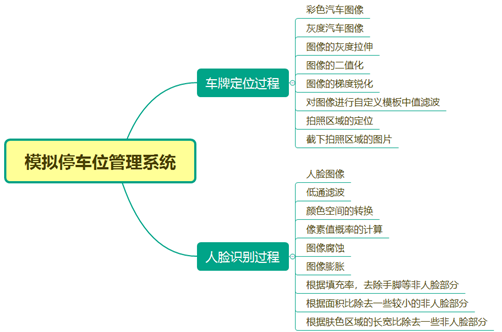功能演示

（一）运行程序时弹出欢迎图片，持续时间为3秒。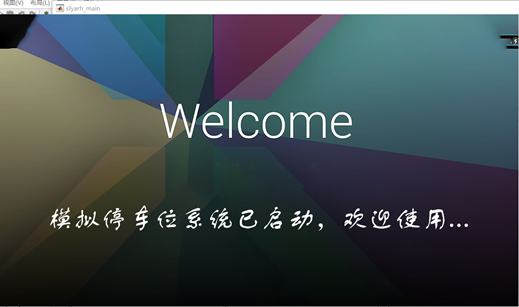（二）待机情况下仅显示如图的组件。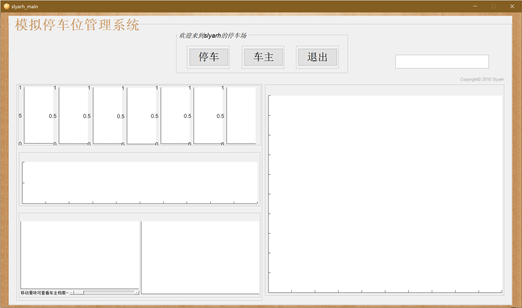（三）车牌识别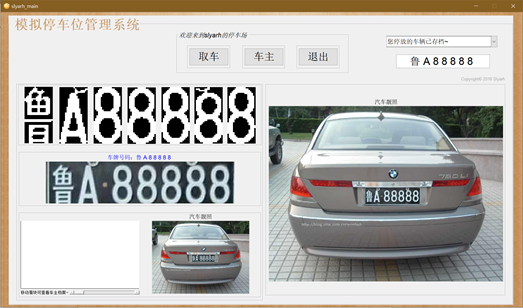（四）下拉菜单可查看处理过程的图片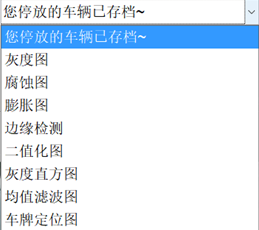（五）人脸定位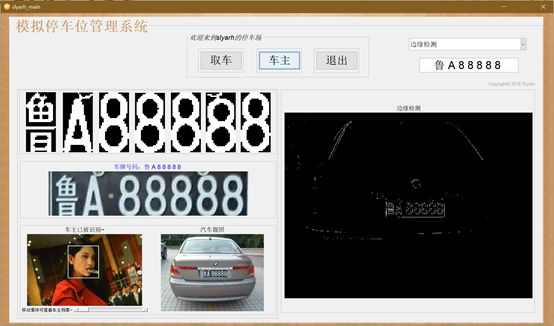（六）移动滑块可查看处理过程的图片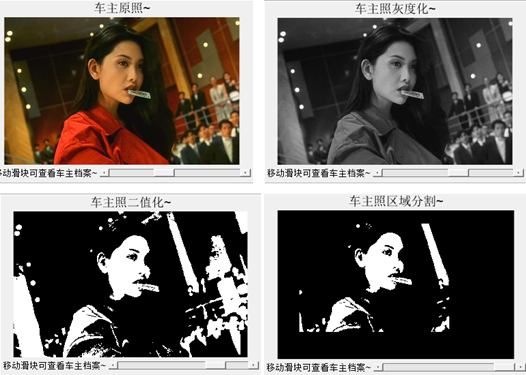代码部分

参考博客：https://blog.csdn.net/zhangquan2015/article/details/78994551

https://blog.csdn.net/u014571132/article/details/51569053

PS：建立如下的几个文件夹，然后把代码都放在同样的目录下。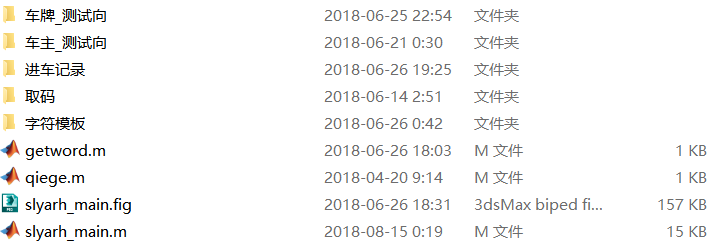测试图以及字符模板在百度云里（密码: etgs）：https://pan.baidu.com/s/1Lu6X1hNfoAede-qrPNkTiQ

下面是程序了。

slyarh_main.m（主程序）

function varargout = slyarh_main(varargin)
gui_Singleton = 1;
gui_State = struct('gui_Name',       mfilename, ...
'gui_Singleton',  gui_Singleton, ...
'gui_OpeningFcn', @slyarh_main_OpeningFcn, ...
'gui_OutputFcn',  @slyarh_main_OutputFcn, ...
'gui_LayoutFcn',  [] , ...
'gui_Callback',   []);
if nargin && ischar(varargin{1})
gui_State.gui_Callback = str2func(varargin{1});
end
if nargout
[varargout{1:nargout}] = gui_mainfcn(gui_State, varargin{:});
else
gui_mainfcn(gui_State, varargin{:});
end
function slyarh_main_OpeningFcn(hObject, eventdata, handles, varargin)
handles.output = hObject;
guidata(hObject, handles);
function varargout = slyarh_main_OutputFcn(hObject, eventdata, handles)
varargout{1} = handles.output;

%“※﹏﹋﹏﹋﹏﹋﹏﹋﹏﹋﹏﹋﹏﹋﹏﹋﹏ 启动画面 ﹏﹋﹏﹋﹏﹋﹏﹋﹏﹋﹏﹋﹏﹋﹏﹋﹏ ※”
ImageFile  = '.\字符模板\wel.png';
ScreenSize = get(0,'ScreenSize');
jfBounds   = num2cell([...
(ScreenSize(3)-jImage.getWidth)/2 ...
(ScreenSize(4)-jImage.getHeight)/2 ...
jImage.getWidth ...
jImage.getHeight]);
jFrame     = javax.swing.JFrame;
icon       = javax.swing.ImageIcon(jImage);
label      = javax.swing.JLabel(icon);
jFrame.setUndecorated(true)
jFrame.setBounds(jfBounds{:});
jFrame.pack
jFrame.show
pause(3)
jFrame.dispose
%“※﹏﹋﹏﹋﹏﹋﹏﹋﹏﹋﹏﹋﹏﹋﹏﹋﹏  ﹏﹋﹏﹋﹏﹋﹏﹋﹏﹋﹏﹋﹏﹋﹏﹋﹏ ※”

%“※﹏﹋﹏﹋﹏﹋﹏﹋﹏﹋﹏﹋﹏﹋﹏﹋﹏ 背景和logo ﹏﹋﹏﹋﹏﹋﹏﹋﹏﹋﹏﹋﹏﹋﹏﹋﹏ ※”
h = handles.figure1; %创建一个Figure，并返回其句柄
newIcon = javax.swing.ImageIcon('.\字符模板\logo.png')
figFrame = get(h,'JavaFrame'); %取得Figure的JavaFrame。
figFrame.setFigureIcon(newIcon); %修改图标
ha=axes('units','normalized','pos',[0 0 1 1]);
uistack(ha,'down');
%设置程序的背景图为beijing1.jpg
image(ii);
colormap gray
set(ha,'handlevisibility','off','visible','off');
%“※﹏﹋﹏﹋﹏﹋﹏﹋﹏﹋﹏﹋﹏﹋﹏﹋﹏  ﹏﹋﹏﹋﹏﹋﹏﹋﹏﹋﹏﹋﹏﹋﹏﹋﹏ ※”

%“※﹏﹋﹏﹋﹏﹋﹏﹋﹏﹋﹏﹋﹏﹋﹏﹋﹏ 停车 ﹏﹋﹏﹋﹏﹋﹏﹋﹏﹋﹏﹋﹏﹋﹏﹋﹏ ※”
function bt_in_Callback(hObject, eventdata, handles)
[filename pathname]=uigetfile({'*.jpg';'*.png';'*.bmp'}, '正在拍摄入场的车辆~');
handles.I=I;
guidata(hObject, handles);
axes(handles.axes1);imshow(I);title('汽车靓照');
axes(handles.axes11);imshow(I);title('汽车靓照');
imwrite(I,'.\进车记录\原.jpg');
set(handles.bt_new,'visible','on');
set(handles.ui_in,'visible','off');
set(handles.ui_out,'visible','on');
%“※﹏﹋﹏﹋﹏﹋﹏﹋﹏﹋﹏﹋﹏﹋﹏﹋﹏ 进车 ﹏﹋﹏﹋﹏﹋﹏﹋﹏﹋﹏﹋﹏﹋﹏﹋﹏ ※”
I=handles.I;
I1=rgb2gray(I);
I2=edge(I1,'roberts',0.18,'both');
imwrite(I1,'.\进车记录\灰度.jpg');
imwrite(I2,'.\进车记录\边缘.jpg');
se=[1;1;1];
I3=imerode(I2,se);%腐蚀操作
imwrite(I2,'.\进车记录\腐蚀.jpg');
se=strel('rectangle',[25,25]);
I4=imclose(I3,se);%图像聚类，填充图像
I5=bwareaopen(I4,2000);%去除聚团灰度值小于2000的部分
[y,x,z]=size(I5);%返回15各维的尺寸，存储在x,y,z中
myI=double(I5);
tic      %tic计时开始，toc结束
Blue_y=zeros(y,1);%产生一个y*1的零针
for i=1:y
for j=1:x
if(myI(i,j,1)==1)%如果myI图像坐标为（i，j）点值为1，即背景颜色为蓝色，blue加一
Blue_y(i,1)=Blue_y(i,1)+1;%蓝色像素点统计
end
end
end
[temp MaxY]=max(Blue_y);
%Y方向车牌区域确定
%temp为向量yellow_y的元素中的最大值，MaxY为该值得索引
PY1=MaxY;
while((Blue_y(PY1,1)>=5)&&(PY1>1))
PY1=PY1-1;
end
PY2=MaxY;
while((Blue_y(PY2,1)>=5)&&(PY2<y))
PY2=PY2+1;
end
IY=I(PY1:PY2,:,:);
%X方向车牌区域确定
Blue_x=zeros(1,x);%进一步确认x方向的车牌区域
for j=1:x
for i=PY1:PY2
if(myI(i,j,1)==1)
Blue_x(1,j)=Blue_x(1,j)+1;
end
end
end
PX1=1;
while((Blue_x(1,PX1)<3)&&(PX1<x))
PX1=PX1+1;
end
PX2=x;
while((Blue_x(1,PX2)<3)&&(PX2>PX1))
PX2=PX2-1;
end
PX1=PX1-1;%对车牌区域的矫正
PX2=PX2+1;
dw=I(PY1:PY2-8,PX1:PX2,:);
t=toc;
imwrite(dw,'.\进车记录\定位.jpg');
b=rgb2gray(dw);%将车牌图像转换为灰度图
g_max=double(max(max(b)));
g_min=double(min(min(b)));
T=round(g_max-(g_max-g_min)/3);%T为二值化的阈值
[m,n]=size(b);
d=(double(b)>=T);%d:二值图像
imwrite(d,'.\进车记录\二值.jpg');
% imwrite(d,'二值化.jpg');
%均值滤波前
%滤波
h=fspecial('average',3);
%建立预定义的滤波算子，average为均值滤波，模板尺寸为3*3
d=im2bw(round(filter2(h,d)));%使用指定的滤波器h对h进行d即均值滤波
imwrite(d,'.\进车记录\均值.jpg');
% imwrite(d,'均值滤波.jpg');
%某些图像进行操作
%膨胀或腐蚀
se=eye(2);%单位矩阵
[m,n]=size(d);%返回信息矩阵
if bwarea(d)/m/n>=0.365%计算二值图像中对象的总面积与整个面积的比是否大于0.365
d=imerode(d,se);%如果大于0.365则进行腐蚀
elseif bwarea(d)/m/n<=0.235%计算二值图像中对象的总面积与整个面积的比值是否小于0.235
d=imdilate(d,se);%%如果小于则实现膨胀操作
end
imwrite(I2,'.\进车记录\膨胀.jpg');
%寻找连续有文字的块，若长度大于某阈值，则认为该块有两个字符组成，需要分割
d=qiege(d);
[m,n]=size(d);
k1=1;
k2=1;
s=sum(d);
j=1;
while j~=n
while s(j)==0
j=j+1;
end
k1=j;
while s(j)~=0 && j<=n-1
j=j+1;
end
k2=j-1;
if k2-k1>=round(n/6.5)
[val,num]=min(sum(d(:,[k1+5:k2-5])));
d(:,k1+num+5)=0;%分割
end
end
%再切割
d=qiege(d);
%切割出7个字符
y1=10;
y2=0.25;
flag=0;
word1=[];
while flag==0
[m,n]=size(d);
left=1;
wide=0;
while sum(d(:,wide+1))~=0
wide=wide+1;
end
if wide<y1 %认为是左干扰
d(:,[1:wide])=0;
d=qiege(d);
else
temp=qiege(imcrop(d,[1 1 wide m]));
[m,n]=size(temp);
all=sum(sum(temp));
two_thirds=sum(sum(temp([round(m/3):2*round(m/3)],:)));
if two_thirds/all>y2
flag=1;word1=temp;%word1
end
d(:,[1:wide])=0;d=qiege(d);
end
end
%分割出第二至七个字符
[word2,d]=getword(d);
[word3,d]=getword(d);
[word4,d]=getword(d);
[word5,d]=getword(d);
[word6,d]=getword(d);
[word7,d]=getword(d);
[m,n]=size(word1);
%商用系统程序中归一化大小为40*20
word1=imresize(word1,[40 20]);
word2=imresize(word2,[40 20]);
word3=imresize(word3,[40 20]);
word4=imresize(word4,[40 20]);
word5=imresize(word5,[40 20]);
word6=imresize(word6,[40 20]);
word7=imresize(word7,[40 20]);
axes(handles.axes2);imshow(word1);
axes(handles.axes3);imshow(word2);
axes(handles.axes4);imshow(word3);
axes(handles.axes5);imshow(word4);
axes(handles.axes6);imshow(word5);
axes(handles.axes7);imshow(word6);
axes(handles.axes8);imshow(word7);
imwrite(word1,'.\取码\1.jpg');
imwrite(word2,'.\取码\2.jpg');
imwrite(word3,'.\取码\3.jpg');
imwrite(word4,'.\取码\4.jpg');
imwrite(word5,'.\取码\5.jpg');
imwrite(word6,'.\取码\6.jpg');
imwrite(word7,'.\取码\7.jpg');
liccode=char(['0':'9' 'A':'Z' '辽粤豫鄂鲁陕京津']);
SubBw2=zeros(40,20);
l=1;
for I=1:7;
ii=int2str(I);
filename=strcat('.\取码\',ii);% 读取子文件夹的名字和路径
SegBw2=imresize(t,[40 20],'nearest');
SegBw2=double(SegBw2)>20;
if l==1 %第一位汉字识别
kmin=37;
kmax=43;
elseif l==2 %第二位字母识别
kmin=11;
kmax=36;
else l>=3   %第三位后字母或数字识别
kmin=1;
kmax=36;
end
for k2=kmin:kmax
fname=strcat('字符模板\',liccode(k2),'.jpg');
SamBw2=double(SamBw2)>1;
for i=1:40
for j=1:20
SubBw2(i,j)=SegBw2(i,j)-SamBw2(i,j);
end
end
%相当于两幅图相减得第三幅图
Dmax=0;
for k1=1:40;
for l1=1:20
if(SubBw2(k1,l1)>0 | SubBw2(k1,l1)<0)
Dmax=Dmax+1;
end
end
end
Error(k2)=Dmax;
end
Error1=Error(kmin:kmax);
MinError=min(Error1);
findc=find(Error1==MinError);
Code(l*2-1)=liccode(findc(1)+kmin-1);
Code(l*2)=' ';
l=l+1;
end
axes(handles.axes9);imshow(dw),title(['车牌号码：',Code],'Color','b');
set(handles.text_out,'String',Code);
imwrite(I1,'.\进车记录\直方.jpg');
%“※﹏﹋﹏﹋﹏﹋﹏﹋﹏﹋﹏﹋﹏﹋﹏﹋﹏  ﹏﹋﹏﹋﹏﹋﹏﹋﹏﹋﹏﹋﹏﹋﹏﹋﹏ ※”

%“※﹏﹋﹏﹋﹏﹋﹏﹋﹏﹋﹏﹋﹏﹋﹏﹋﹏ 取车 ﹏﹋﹏﹋﹏﹋﹏﹋﹏﹋﹏﹋﹏﹋﹏﹋﹏ ※”
function bt_out_Callback(hObject, eventdata, handles)
delete('.\进车记录\*.jpg') % 将删除指定目录上的所有.m文件
axes(handles.axes1);cla reset;
axes(handles.axes2);cla reset;
axes(handles.axes3);cla reset;
axes(handles.axes4);cla reset;
axes(handles.axes5);cla reset;
axes(handles.axes6);cla reset;
axes(handles.axes7);cla reset;
axes(handles.axes8);cla reset;
axes(handles.axes9);cla reset;
axes(handles.axes10);cla reset;
axes(handles.axes11);cla reset;
set(handles.bt_new,'visible','off');
set(handles.ui_in,'visible','on');
set(handles.ui_out,'visible','off');
set(handles.text_out,'String','');
%“※﹏﹋﹏﹋﹏﹋﹏﹋﹏﹋﹏﹋﹏﹋﹏﹋﹏  ﹏﹋﹏﹋﹏﹋﹏﹋﹏﹋﹏﹋﹏﹋﹏﹋﹏ ※”

%“※﹏﹋﹏﹋﹏﹋﹏﹋﹏﹋﹏﹋﹏﹋﹏﹋﹏ 退出 ﹏﹋﹏﹋﹏﹋﹏﹋﹏﹋﹏﹋﹏﹋﹏﹋﹏ ※”
function bt_exit_Callback(hObject, eventdata, handles)
close(gcf);
%“※﹏﹋﹏﹋﹏﹋﹏﹋﹏﹋﹏﹋﹏﹋﹏﹋﹏  ﹏﹋﹏﹋﹏﹋﹏﹋﹏﹋﹏﹋﹏﹋﹏﹋﹏ ※”

%“※﹏﹋﹏﹋﹏﹋﹏﹋﹏﹋﹏﹋﹏﹋﹏﹋﹏ 车主 ﹏﹋﹏﹋﹏﹋﹏﹋﹏﹋﹏﹋﹏﹋﹏﹋﹏ ※”
function bt_peo_Callback(hObject, eventdata, handles)
% 载入图像
[filename pathname]=uigetfile({'*.jpg';'*.png';'*.bmp'}, '请拍摄车主照~');
%handles.Img=Img;
guidata(hObject, handles);
imwrite(Img,'.\进车记录\车主.jpg');
%axes(handles.axes10);imshow(I);title('汽车靓照');
if ndims(Img) == 3
I=rgb2gray(Img);
else
I = Img;
end
BW = im2bw(I, graythresh(I)); % 二值化
imwrite(I,'.\进车记录\车主灰度.jpg');
imwrite(BW,'.\进车记录\车主二值.jpg');
[n1, n2] = size(BW);
r = floor(n1/10); % 分成10块，行
c = floor(n2/10); % 分成10块，列
x1 = 1; x2 = r; % 对应行初始化
s = r*c; % 块面积
for i = 1:11
y1 = 1; y2 = c; % 对应列初始化
for j = 1:11
if (y2<=c || y2>=9*c) || (x1==1 || x2==r*10)
% 如果是在四周区域
BW(x1:x2, y1:y2) = 0;
end
y1 = y1+c; % 列跳跃
y2 = y2+c; % 列跳跃
end
x1 = x1+r; % 行跳跃
x2 = x2+r; % 行跳跃
end
imwrite(BW,'.\进车记录\车主识别.jpg');
[L, num] = bwlabel(BW, 8); % 区域标记
stats = regionprops(L, 'BoundingBox'); % 得到包围矩形框
Bd = cat(1, stats.BoundingBox);
[s1, s2] = size(Bd);
mx = 0;
for k = 1:s1
p = Bd(k, 3)*Bd(k, 4); % 宽*高
if p>mx && (Bd(k, 3)/Bd(k, 4))<1.8
% 如果满足面积块大，而且宽/高<1.8
mx = p;
j = k;
end
end
axes(handles.axes10);imshow(Img);%hold on;
rectangle('Position', Bd(j, :), ...
'EdgeColor', 'w', 'LineWidth', 1);title('车主已被识别~');

%“※﹏﹋﹏﹋﹏﹋﹏﹋﹏﹋﹏﹋﹏﹋﹏﹋﹏  ﹏﹋﹏﹋﹏﹋﹏﹋﹏﹋﹏﹋﹏﹋﹏﹋﹏ ※”

%“※﹏﹋﹏﹋﹏﹋﹏﹋﹏﹋﹏﹋﹏﹋﹏﹋﹏ 查看属性 ﹏﹋﹏﹋﹏﹋﹏﹋﹏﹋﹏﹋﹏﹋﹏﹋﹏ ※”
function bt_new_Callback(hObject, eventdata, handles)
val=get(handles.bt_new,'value');
switch val
case 1
axes(handles.axes1);imshow(pic_show);title('汽车靓照');
case 2
axes(handles.axes1);imshow(pic_show);title('灰度图');
case 3
axes(handles.axes1);imshow(pic_show);title('腐蚀化');
case 4
axes(handles.axes1);imshow(pic_show);title('膨胀化');
case 5
axes(handles.axes1);imshow(pic_show);title('边缘检测');
case 6
axes(handles.axes1);imshow(pic_show);title('二值化');
case 7
axes(handles.axes1);imhist(pic_show);title('灰度化直方图');
case 8
axes(handles.axes1);imshow(pic_show);title('均值滤波处理');
case 9
axes(handles.axes1);imshow(pic_show);title('车牌定位');
end
function bt_new_CreateFcn(hObject, eventdata, handles)%构造
%“※﹏﹋﹏﹋﹏﹋﹏﹋﹏﹋﹏﹋﹏﹋﹏﹋﹏  ﹏﹋﹏﹋﹏﹋﹏﹋﹏﹋﹏﹋﹏﹋﹏﹋﹏ ※”

%“※﹏﹋﹏﹋﹏﹋﹏﹋﹏﹋﹏﹋﹏﹋﹏﹋﹏ 滑块 ﹏﹋﹏﹋﹏﹋﹏﹋﹏﹋﹏﹋﹏﹋﹏﹋﹏ ※”
function hua_peo_Callback(hObject, eventdata, handles)
p = get(handles.hua_peo, 'Value');
if(p>=0 && p<=0.2)
if ndims(peo_show) == 3
I=rgb2gray(peo_show);
else
I = peo_show;
end
BW = im2bw(I, graythresh(I)); % 二值化
[n1, n2] = size(BW);
r = floor(n1/10); % 分成10块，行
c = floor(n2/10); % 分成10块，列
x1 = 1; x2 = r; % 对应行初始化
s = r*c; % 块面积
for i = 1:11
y1 = 1; y2 = c; % 对应列初始化
for j = 1:11
if (y2<=c || y2>=9*c) || (x1==1 || x2==r*10)
% 如果是在四周区域
BW(x1:x2, y1:y2) = 0;
end
y1 = y1+c; % 列跳跃
y2 = y2+c; % 列跳跃
end
x1 = x1+r; % 行跳跃
x2 = x2+r; % 行跳跃
end
[L, num] = bwlabel(BW, 8); % 区域标记
stats = regionprops(L, 'BoundingBox'); % 得到包围矩形框
Bd = cat(1, stats.BoundingBox);
[s1, s2] = size(Bd);
mx = 0;
for k = 1:s1
p = Bd(k, 3)*Bd(k, 4); % 宽*高
if p>mx && (Bd(k, 3)/Bd(k, 4))<1.8 % 如果满足面积块大，而且宽/高<1.8
mx = p;
j = k;
end
end
axes(handles.axes10);imshow(peo_show);
rectangle('Position', Bd(j, :), ...
'EdgeColor', 'w', 'LineWidth', 1);title('车主已识别~');
elseif(p>0.2 && p<=0.4)
axes(handles.axes10);imshow(peo_show);title('车主原照~');
elseif(p>0.4 && p<=0.6)
axes(handles.axes10);imshow(peo_show);title('车主照灰度化~');
elseif(p>0.6 && p<=0.8)
axes(handles.axes10);imshow(peo_show);title('车主照二值化~');
else
axes(handles.axes10);imshow(peo_show);title('车主照区域分割~');
end

function hua_peo_CreateFcn(hObject, eventdata, handles)
%“※﹏﹋﹏﹋﹏﹋﹏﹋﹏﹋﹏﹋﹏﹋﹏﹋﹏  ﹏﹋﹏﹋﹏﹋﹏﹋﹏﹋﹏﹋﹏﹋﹏﹋﹏ ※”

if ispc && isequal(get(hObject,'BackgroundColor'), get(0,'defaultUicontrolBackgroundColor'))
set(hObject,'BackgroundColor','white');
end
if isequal(get(hObject,'BackgroundColor'), get(0,'defaultUicontrolBackgroundColor'))
set(hObject,'BackgroundColor',[.9 .9 .9]);
end
if isequal(get(hObject,'BackgroundColor'), get(0,'defaultUicontrolBackgroundColor'))
set(hObject,'BackgroundColor',[.9 .9 .9]);
end

function uipanel1_CreateFcn(hObject, eventdata, handles)

function text_out_Callback(hObject, eventdata, handles)

function text_out_CreateFcn(hObject, eventdata, handles)

if ispc && isequal(get(hObject,'BackgroundColor'), get(0,'defaultUicontrolBackgroundColor'))
set(hObject,'BackgroundColor','white');
end

qiege.m（子程序1）

function e=qiege(d)
[m,n]=size(d);
top=1;
bottom=m;
left=1;
right=n;
while sum(d(top,:))==0 && top<=m
top=top+1;
end
while sum(d(bottom,:))==0 && bottom>1
bottom=bottom-1;
end
while sum(d(:,left))==0 && left<n
left=left+1;
end
while sum(d(:,right))==0 && right>=1
right=right-1;
end
dd=right-left;
hh=bottom-top;
e=imcrop(d,[left top dd hh]);

getword.m（子程序2）

function[word,result]=getword(d)
word=[];
flag=0;
y1=8;
y2=0.5;
while flag==0
[m,n]=size(d);
wide=0;
while sum(d(:,wide+1))~=0 && wide<=n-2
wide=wide+1;
end
temp=qiege(imcrop(d,[1 1 wide m]));%用于返回图像的一个裁剪区域
[m1,n1]=size(temp);
if wide<y1 && n1/m1>y2
d(:,[1:wide])=0;
if sum(sum(d))~=0
d=qiege(d);%切割出最小范围
else word=[];flag=1;
end
else
word=qiege(imcrop(d,[1 1 wide m]));
d(:,[1:wide])=0;
if sum(sum(d))~=0
d=qiege(d);
flag=1;
else d=[];
end
end
end
result=d;

✎﹏﹏₯㎕《晴天》童年的荡秋千...﹍﹍﹍﹍﹍﹍
展开全文matlab 数字图像处理
• 本文学习资源来自《机器学习实践指南 案例应用解析》 人脸辨识生物特征识别(BIOMETRICS) 技术，是指通过计算机利用人体所固有的...人脸定位OpenCV的接口可以实现人脸定位。cvHaarDetectObjects是opencv1中的函数，open
*本文学习资源来自《机器学习实践指南 案例应用解析》 *
人脸辨识
生物特征识别(BIOMETRICS) 技术，是指通过计算机利用人体所固有的生理特征（指纹、虹膜、面相、DNA等）或行为特征(步态、击键习惯等)来进行个人身份鉴定的技术。
——百度百科
人脸识别属于生物特征识别技术中的一种。
人脸定位
OpenCV的接口可以实现人脸定位。
cvHaarDetectObjects是opencv1中的函数，opencv2中人脸检测使用的是 detectMultiScale函数。它可以检测出图片中所有的人脸，并将人脸用vector保存各个人脸的坐标、大小（用矩形表示），函数由分类器对象调用：
void detectMultiScale(
const Mat& image,
CV_OUT vector<Rect>& objects,
double scaleFactor = 1.1,
int minNeighbors = 3,
int flags = 0,
Size minSize = Size(),
Size maxSize = Size()
);

参数1：image–待检测图片，一般为灰度图像加快检测速度；
参数2：objects–被检测物体的矩形框向量组；
参数3：scaleFactor–表示在前后两次相继的扫描中，搜索窗口的比例系数。默认为1.1即每次搜索窗口依次扩大10%;
参数4：minNeighbors–表示构成检测目标的相邻矩形的最小个数(默认为3个)。
如果组成检测目标的小矩形的个数和小于 min_neighbors - 1 都会被排除。
如果min_neighbors 为 0, 则函数不做任何操作就返回所有的被检候选矩形框，
这种设定值一般用在用户自定义对检测结果的组合程序上；
参数5：flags–要么使用默认值，要么使用CV_HAAR_DO_CANNY_PRUNING，如果设置为
CV_HAAR_DO_CANNY_PRUNING，那么函数将会使用Canny边缘检测来排除边缘过多或过少的区域，
因此这些区域通常不会是人脸所在区域；
参数6、7：minSize和maxSize用来限制得到的目标区域的范围。
代码
首先，下载OpenCV库：
https://github.com/opencv/opencv
代码1：
# -*- coding: utf-8 -*-
# coding=utf-8
import cv2

OPCV_PATH=r"D:/tools/opencv/sources"
color = (0, 0, 0)  # 设置人脸框的颜色

def findface(src):
image = cv2.cvtColor(src, cv2.COLOR_BGR2GRAY)
cv2.equalizeHist(image, image)  # 灰度图像进行直方图等距化

# 加载OpenCv的面部特征库
# 找到人脸的位置
# 设定最小图像的大小
divisor = 8
h = image.shape
w = image.shape
minSize = (int(w / divisor), int(h / divisor))  # 这里加了一个取整函数
faceRects = classfier.detectMultiScale(image, 1.2, 2, cv2.CASCADE_SCALE_IMAGE, minSize)

if len(faceRects) > 0:  # 如果人脸数组长度大于0
for faceRect in faceRects:  # 对每一个人脸画矩形框
x, y, w, h = faceRect
cv2.rectangle(image, (x, y), (x + w, y + h), color)
cv2.imshow('img',image)

fn = 'test2.jpg'

findface(my_img)

cv2.waitKey()
cv2.destroyAllWindows()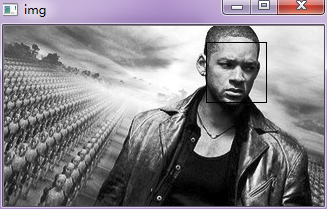代码2：
# -*- coding: utf-8 -*-
# coding=utf-8

import cv2

# 待检测的图片路径
OPCV_PATH=r"D:/tools/opencv/sources"
imagepath = r'./test1.jpg'

# 加载面部特征库

# 读取图片
gray = cv2.cvtColor(image, cv2.COLOR_BGR2GRAY)

# 探测图片中的人脸
print ("发现{0}个人脸!".format(len(faces)))

for (x, y, w, h) in faces:
cv2.circle(image, (int((x + x + w) / 2), int((y + y + h) / 2)), int(w / 2), (0, 255, 0), 2)

cv2.imshow("Find Faces!", image)
cv2.waitKey(0)

[外链图片转存中…(img-uKpaAtzc-1574725803368)]
参考：
http://blog.sina.com.cn/s/blog_5562b0440102uw7g.html
http://blog.csdn.net/itismelzp/article/details/50379359
http://www.cnblogs.com/hanson1/p/7105265.html


展开全文• 数字图像处理技术与人脸识别 电子科技大学 格拉斯哥学院 2017/2018级 耿逸飞 2017200602025 序言 如今，随着科技的迅速发展，各大手机厂商对智能手机的研发也愈发深入。传统的手机解锁方式已经不足以满足各大手机...
数字图像处理技术与人脸识别
电子科技大学 格拉斯哥学院 2017/2018级 耿逸飞 2017200602025
序言
如今，随着科技的迅速发展，各大手机厂商对智能手机的研发也愈发深入。传统的手机解锁方式已经不足以满足各大手机厂商以及手机用户们，诸如华为、苹果等手机生产商早已将目光投向更加新颖的技术——人脸识别。顾名思义，人脸识别就是通过对每张脸的不同特征的辨识来实现对不同个体的脸的识别。尽管人们在人脸识别领域已经有了显著的成果，但是人脸识别技术依然有许多值得研究，更进一步的地方。
一、人脸识别概要
人脸识别作为一个很受欢迎的研究领域，有着自己的独到之处：
1、人脸识别的两大主要用途：
a.识别身份；
b.验证身份
2、人脸识别的重要性：
a.人脸识别是一种容易实现，更为人熟悉的生物特征识别方式;
b.人脸识别是人类视觉最杰出的能力之一，正是人脸识别这一功能，使得人类可以清楚地分辨不同的人之间的差别。
二、人脸识别的主要过程
人脸识别主要包括以下几个方面：人脸的检测与定位、人脸图像的预处理、人脸特征提取、分类识别等。
人脸的检测与定位：从摄像头拍摄的场景中检测出人脸的存在，并从中分理出人脸的区域。
人脸图像的预处理：矫正人脸的尺度、光照（明暗）、旋转（水平垂直）等，由此，程序可以得到一个较为标准、规范化的人脸图像。
人脸特征提取：从经过预处理后的人脸图像中提取出人脸上具有代表性的特征信息，并用计算机语言进行描述。
分类识别：根据前一步提取出的人脸特征信息，将这些信息与数据库中已获得的人脸信息进行比较，找出数据库中特征信息与该待测人脸的特征信息最相似的人脸。
基于人脸识别的用途与研究现状，人脸特征提取与分类识别是人脸识别中最重要的两步。
三、用计算机进行人脸识别的算法
目前，经过研究，研究人员提出了近十种用于人脸识别的算法。这里，我将主要介绍特征脸方法（Eigenface或PCA：principal component analysis基于主成分分析）。
特征子脸技术的基本思想是：从统计的观点，寻找人脸图像分布的基本元素，即人脸图像样本集协方差矩阵的特征向量，以此近似地表征人脸图像。这些特征向量称为特征脸(Eigenface)。
实际上，特征脸反映了隐含在人脸样本集合内部的信息和人脸的结构关系。将眼睛、面颊、下颌的样本集协方差矩阵的特征向量称为特征眼、特征颌和特征唇，统称特征子脸。特征子脸在相应的图像空间中生成子空间，称为子脸空间。计算出测试图像窗口在子脸空间的投影距离，若窗口图像满足阈值比较条件，则判断其为人脸。
基于特征分析的方法，也就是将人脸基准点的相对比率和其它描述人脸脸部特征的形状参数或类别参数等一起构成识别特征向量，这种基于整体脸的识别不仅保留了人脸部件之间的拓扑关系，而且也保留了各部件本身的信息，而基于部件的识别则是通过提取出局部轮廓信息及灰度信息来设计具体识别算法。现在Eigenface(PCA)算法已经与经典的模板匹配算法一起成为测试人脸识别系统性能的基准算法；而自1991年特征脸技术诞生以来，研究者对其进行了各种各样的实验和理论分析，FERET’96测试结果也表明，改进的特征脸算法是主流的人脸识别技术，也是具有最好性能的识别方法之一。
该方法是先确定眼虹膜、鼻翼、嘴角等面像五官轮廓的大小、位置、距离等属性，然后再计算出它们的几何特征量，而这些特征量形成一描述该面像的特征向量。其技术的核心实际为“局部人体特征分析”和“图形/神经识别算法。”这种算法是利用人体面部各器官及特征部位的方法。如对应几何关系多数据形成识别参数与数据库中所有的原始参数进行比较、判断与确认。Turk和Pentland提出特征脸的方法，它根据一组人脸训练图像构造主元子空间，由于主元具有脸的形状，也称为特征脸  ,识别时将测试  图像投影到主元子空间上，得到一组投影系数，和各个已知人的人脸图像比较进行识别。Pentland等报告了相当好的结果，在 200个人的 3000幅图像中得到 95%的正确识别率，在ＦＥＲＥＴ数据库上对 150幅正面人脸象只有一个误识别。但系统在进行特征脸方法之前需要作大量预处理工作如归一化等。
在传统特征脸的基础上，研究者注意到特征值大的特征向量 (即特征脸 )并不一定是分类性能好的方向，据此发展了多种特征 (子空间 )选择方法，如Peng的双子空间方法、Weng的线性歧义分析方法、Belhumeur的FisherFace方法等。事实上，特征脸方法是一种显式主元分析人脸建模，一些线性自联想、线性压缩型ＢＰ网则为隐式的主元分析方法，它们都是把人脸表示为一些向量的加权和，这些向量是训练集叉积阵的主特征向量，Valentin对此作了详细讨论。总之，特征脸方法是一种简单、快速、实用的基于变换系数特征的算法。但是，由于它在本质上依赖于训练集和测试集图像的灰度相关性，而且要求测试图像与训练集比较像，所以它有着很大的局限性。
四、人脸识别技术的不足与发展方向
虽然，经过了数年的发展，人脸识别技术已经日渐成熟但仍然有许多不足以及技术上的难点：
1、光照
由于各种客观条件，我们无法保证在每次进行人脸识别的时候具有相同的光照条件，而不同强度的光线会导致在获取人脸时图像时成像与数据库中已保存的人脸信息差距较大，进而导致无法识别。解决该光照问题主要有三种方法：获取实时光照参数、进行光照补偿、灰度预处理等。但是，由于获取实时光照参数与进行光照补偿两种方法操作麻烦、难度高而且工作量大，所以不适合进行大量应用。还需要继续研究，寻找更优的解决办法。
2、人脸的状态（姿势、表情等）
人的面部表情千变万化，进行人脸识别时很难做到让被识别个体的面部处于与数据库完全相同的表情与姿势。人脸在现实中是一个三维物体，而成像以后的人脸图像是一个二维图像，所以，识别过程中头部的俯仰、脸部的表情势必会遮挡住或扭曲某些重要的人脸特征信息，从而影响人脸识别的进行。虽然，研究人员常用姿态补偿、姿态估计、表情估计等方法来解决这些问题，但是，这些方法都不能完美地克服这些问题。为了可以彻底解决这些问题，完善人脸识别技术，还需要更多的研究。
3、缺少人脸资源
由于人脸识别的研究从近几年才开始兴起，研究人员没有足够庞大的数据库用于研究，更是远远达不到投入实际运用的标准。
五、总结
人脸识别的方法很多，但是各自都有各自的不足之处，博采众长、克服局限性才能让人脸识别技术更上一层楼。若想使人脸识别技术更加完善，还需要努力研究。
六、参考文献
算法学习者的CSDN博客：https://blog.csdn.net/amds123/article/details/72742578
刘世伟 数字图像处理 人脸识别：https://wenku.baidu.com/view/77aed9fda21614791611287a.html


展开全文• 一般来说，人脸识别系统包括图像摄取、人脸定位图像预处理、以及人脸识别（身份确认或者身份查找）。系统输入一般是一张或者一系列含有未确定身份的人脸图像，以及人脸数据库中的若干已知身份的人脸识别。 人脸...
https://www.toutiao.com/a6651763844760732164/

2019-01-29 12:00:57

识别算法

一般来说，人脸识别系统包括图像摄取、人脸定位、图像预处理、以及人脸识别（身份确认或者身份查找）。系统输入一般是一张或者一系列含有未确定身份的人脸图像，以及人脸数据库中的若干已知身份的人脸识别。

人脸图象或者相应的编码，而其输出则是一系列相似度得分，表明待识别的人脸的身份。人脸识别算法分类

基于人脸特征点的识别算法（Feature-based recognition algorithms）。

基于整幅人脸图像的识别算法（Appearance-based recognition algorithms）。

基于模板的识别算法（Template-based recognition algorithms）。

利用神经网络进行识别的算法（Recognition algorithms using neural network）。基于光照估计模型理论

提出了基于Gamma灰度矫正的光照预处理方法,并且在光照估计模型的基础上，进行相应的光照补偿和光照平衡策略。

优化的形变统计校正理论

基于统计形变的校正理论，优化人脸姿态；神经网络识别

强化迭代理论

强化迭代理论是对DLFA人脸检测算法的有效扩展；

独创的实时特征识别理论

该理论侧重于人脸实时数据的中间值处理，从而可以在识别速率和识别效能之间，达到最佳的匹配效果识别数据

人脸识别需要积累采集到的大量人脸图像相关的数据，用来验证算法，不断提高识别准确性，这些数据诸如A Neural Network Face Recognition Assignment(神经网络人脸识别数据)、orl人脸数据库、麻省理工学院生物和计算学习中心人脸识别数据库、埃塞克斯大学计算机与电子工程学院人脸识别数据等。
展开全文人脸识别 图像预处理
• ## 图像处理-人脸识别

千次阅读 2019-07-19 09:59:40
• 针对人脸识别的预处理,采用图像处理技术解决了人脸检测问题。首先建立输入图像的肤色模型,然后进行开运算处理,以消除图像噪声利于后面的眼睛定位。再对二值图像做灰度投影实现人脸粗分割,定位双眼。最后对细化分割...
• 此程序是基于matlab的仿真 共有五个程序 程序都是好使的 没有错误 只要在电脑F盘中存入一张 名为"face3.jpg‘再 运行程序 即可好使 此程序用于人脸图像处理 检测 和定位 其中人脸处理包括图形转换 灰度图像直方图...matlab源程序
• 眼睛定位 根据眼睛定位的流程，一般分为以下几步：眼睛区域初定位，噪声去除，...而且图像处理领域，凡是涉及到阈值的，都是比较头疼的问题（除非那种阈值很明显的问题）。所以，这里尽量避免直接求阈值，来确人脸识别 图像处理 人工智能
•   2019-01-08 16:48:06 随着大数据时代的到来，“人脸”也将成为数据的一部分，人脸识别如何实现？...随着计算机技术以及光学成像技术的发展，集成了人工智能、机器学习、视频图像处理等技术的人脸识...人脸识别
• 过程概要训练一个能识别一张227*227的图像是否是人脸的二分类模型（使用AlexNet网络） =>人脸 =>非人脸 修改训练好的网络模型，数据层改为输入层，全链接层改为全卷积层（起到窗口滑动的作用） 将输入的图片进行...脸部识别 机器学习
• 对RGB图像通过形态学图像处理算法选定区域，再进行细化算法，找到其五官坐标并提取出来；识别率达到85.23%以上。此系统需要大量的前处理和精细的参数选择，对计算量要求较大。 课题采用自建的照片库，取材于摄影...
• 过程：本系列包括从人脸检测、人脸关键点检测、人脸优选、人脸对齐、再到人脸特征提取的一系系列过程，每个模块都有尝试一些网络结构和方法，因为自己主要部署在嵌入式平台、移动端等设备，所以都是采用轻量级的网络...
• 来源 | AI 算法与图像处理 封图 | CSDN 付费下载于视觉中国 本文的内容预览 人脸检测的概念 人脸检测是一种在多种应用中使用的计算机技术，可以识别数字图像中的人脸。人脸检测还指人类在视觉场景中定位人脸的...
• 利用灰度积分垂直和水平投影曲线包含的信息对人脸区域粗定位,并缩小图像处理范围。在得到大致人脸范围的基础上，使用Susan算子定位两个眼角点从而实现眼睛的精确定位。以粗定位为基准,按照适当的方法对鼻、嘴的特征...
• 数字图像处理应用领域 图像是人类获取和交换信息的主要来源，因此，图像处理的应用领域必然涉及到人类生活和工作的方方面面。随着人类活动范围的不断扩大，图像处理的应用领域也将随之不断扩大。 1）航天和航空方面 ...
• 基于opencv的人脸识别，然后进行图像处理从而瞳孔准确定位
• 很多方法都是基于在提取人脸特征点的基础上完成的，然后利用网格模型或者形变模型对特征点进行分析和检测以及分类的方法实现对人脸表情的识别，可以看出人脸关键点（特征点）的提取与定位人脸表情识别占有很重要的...